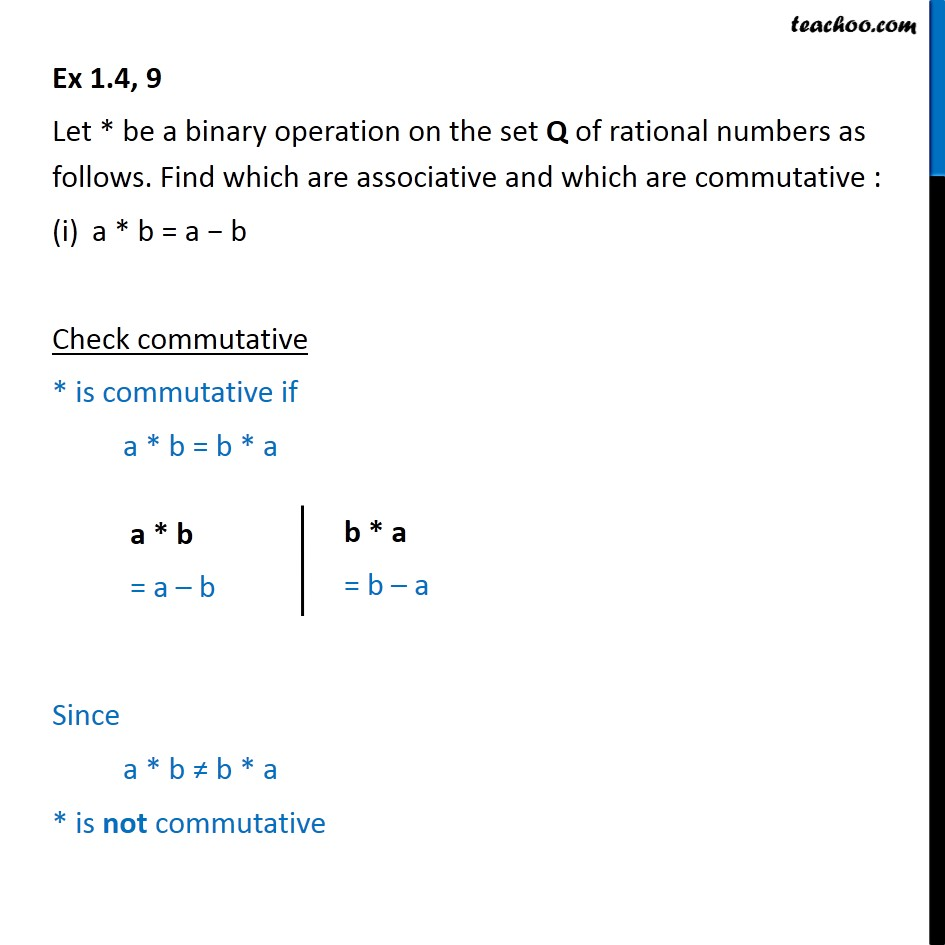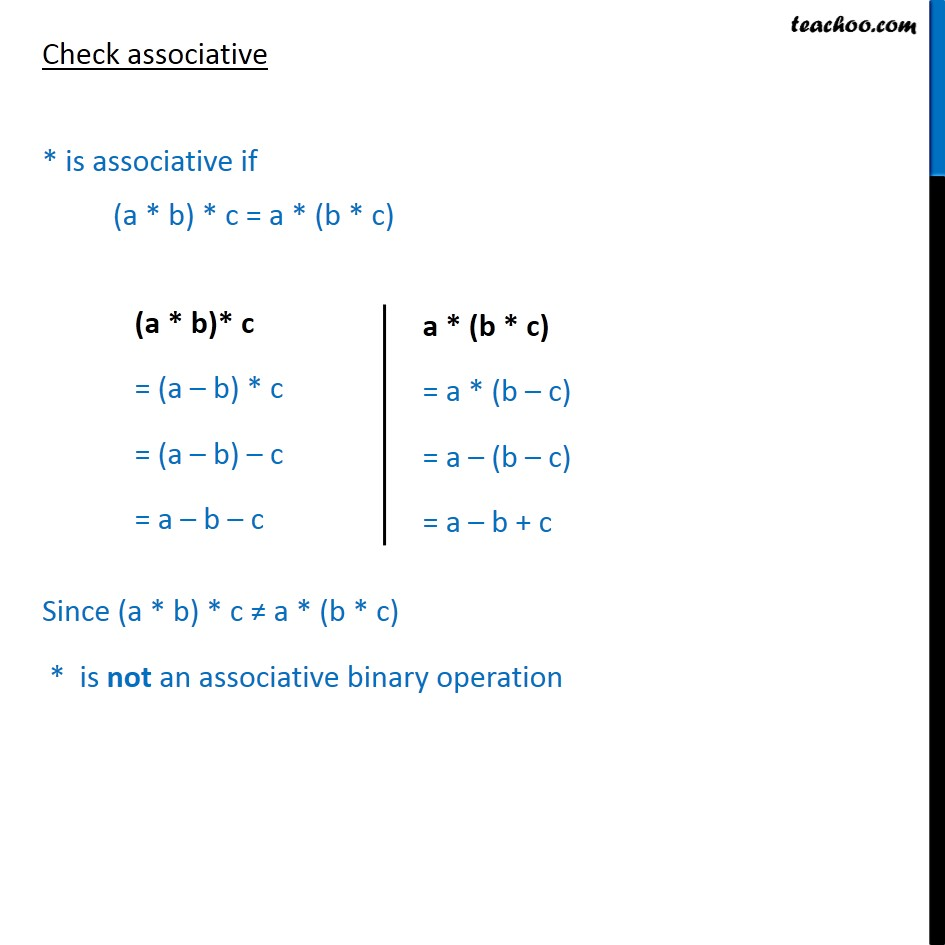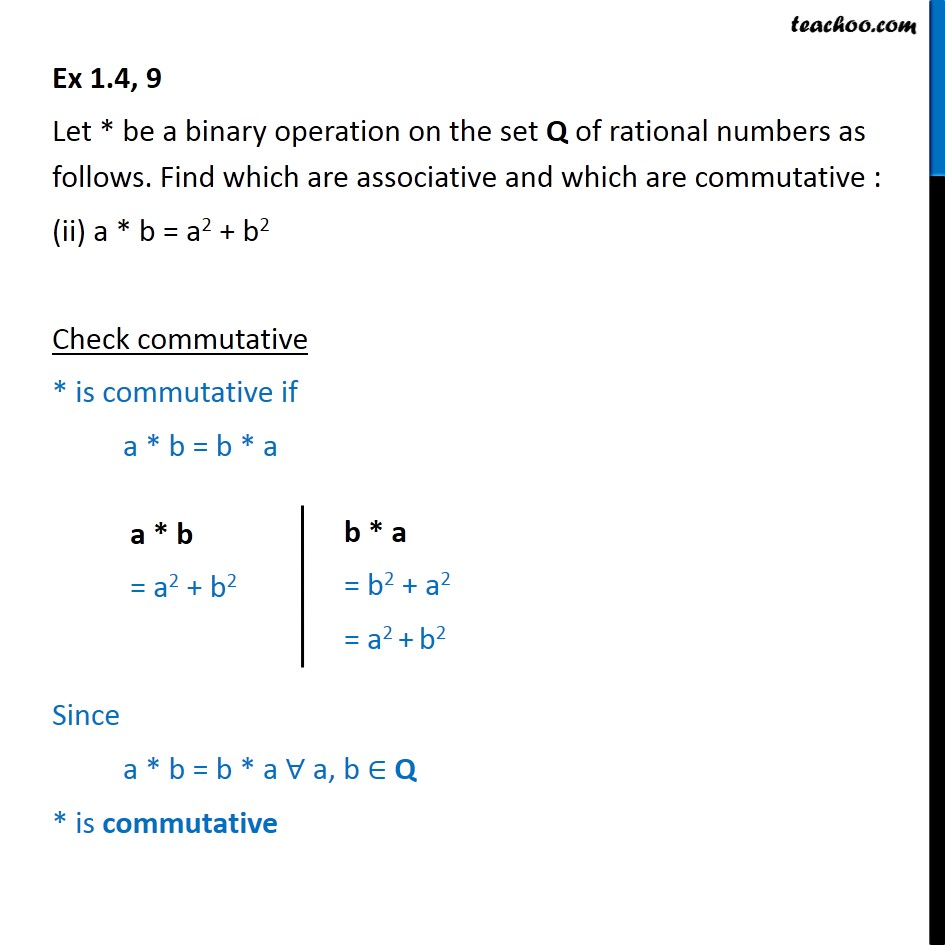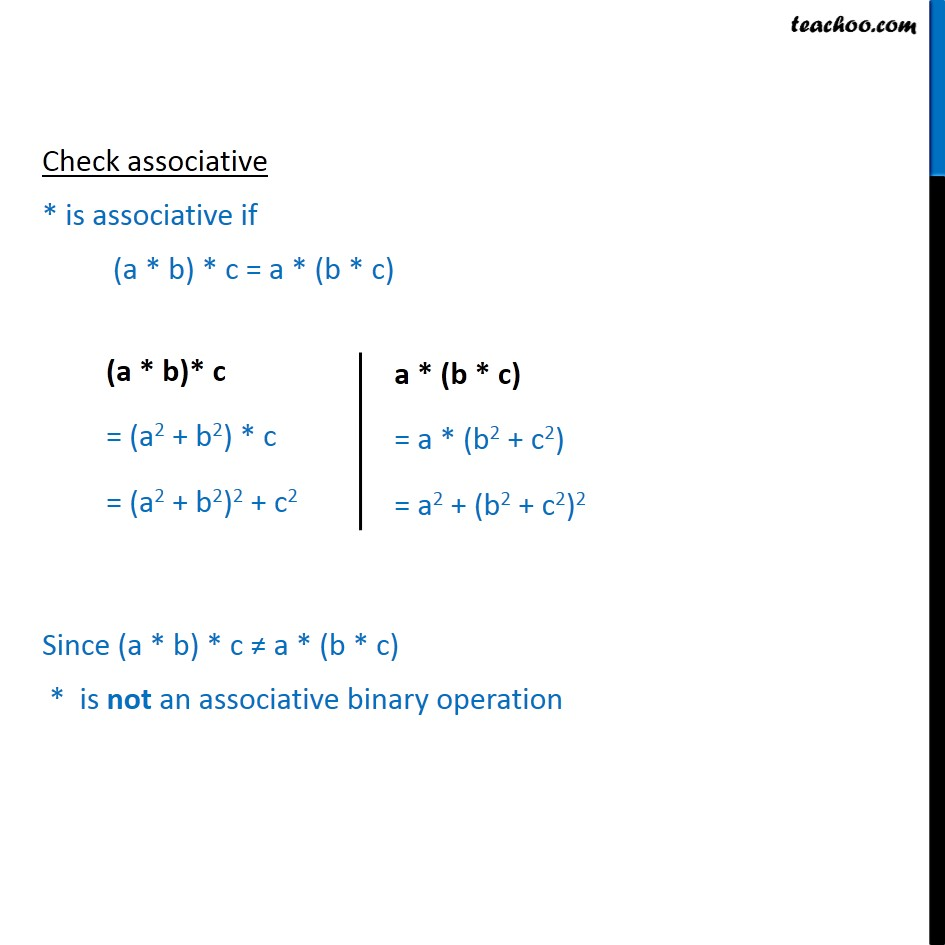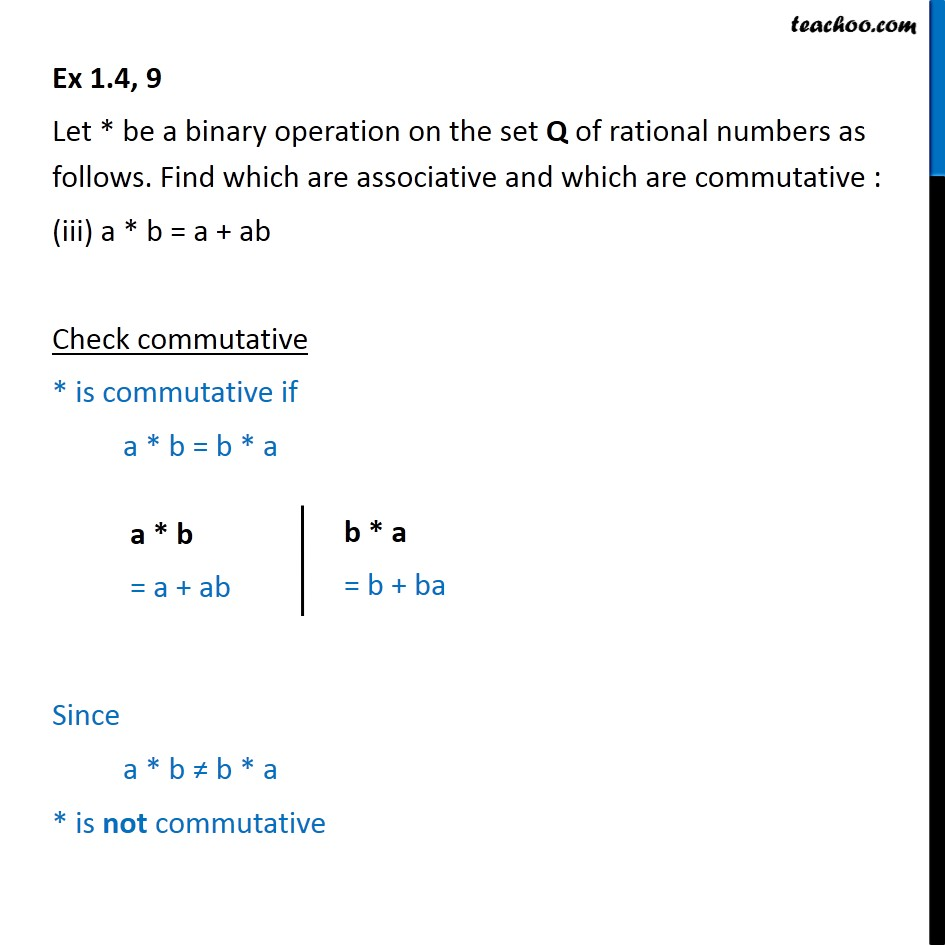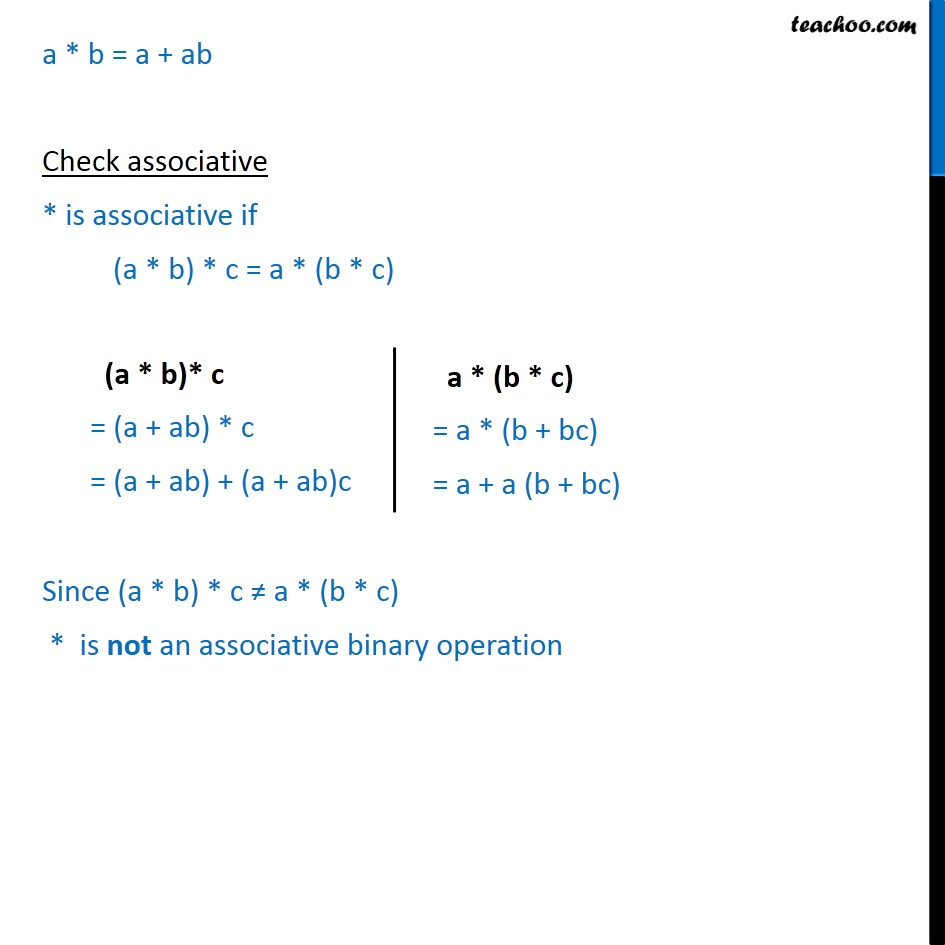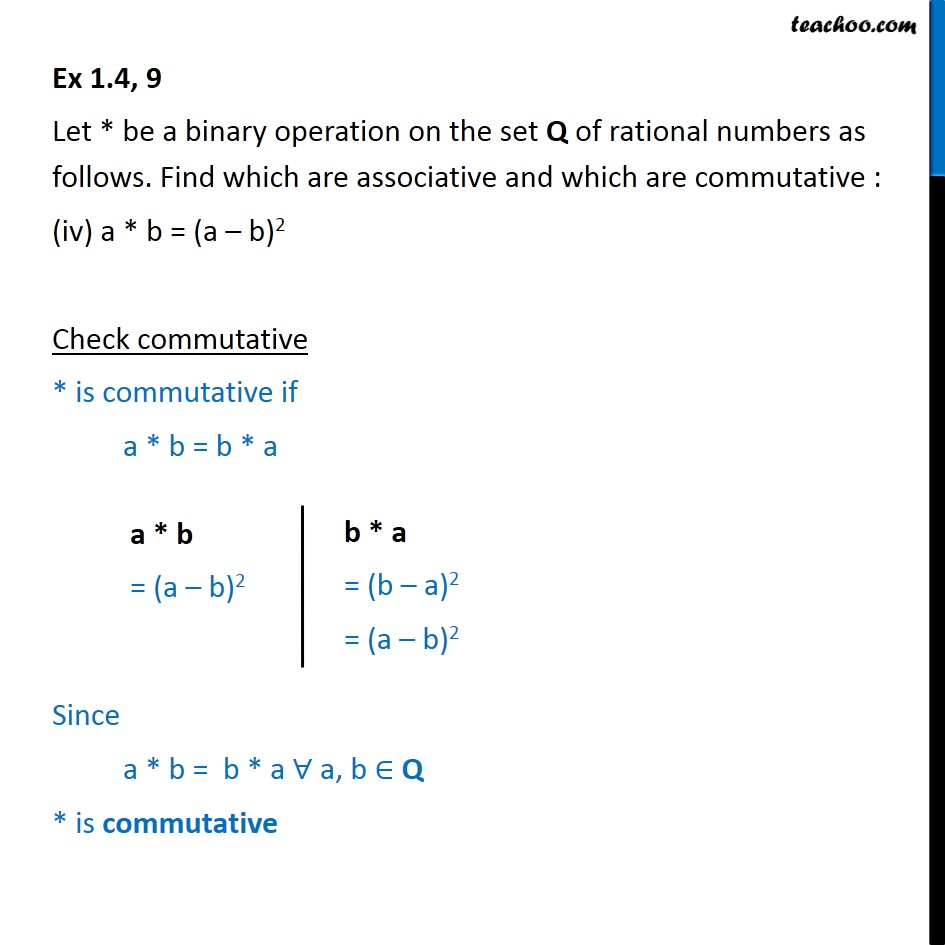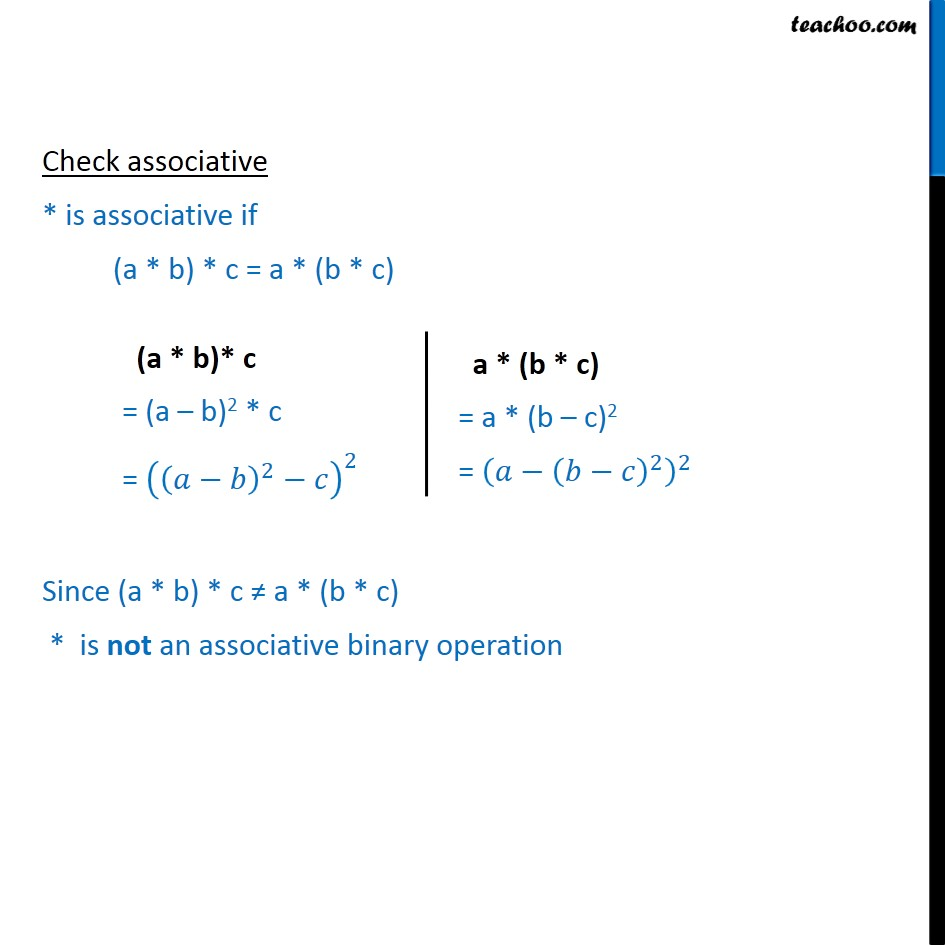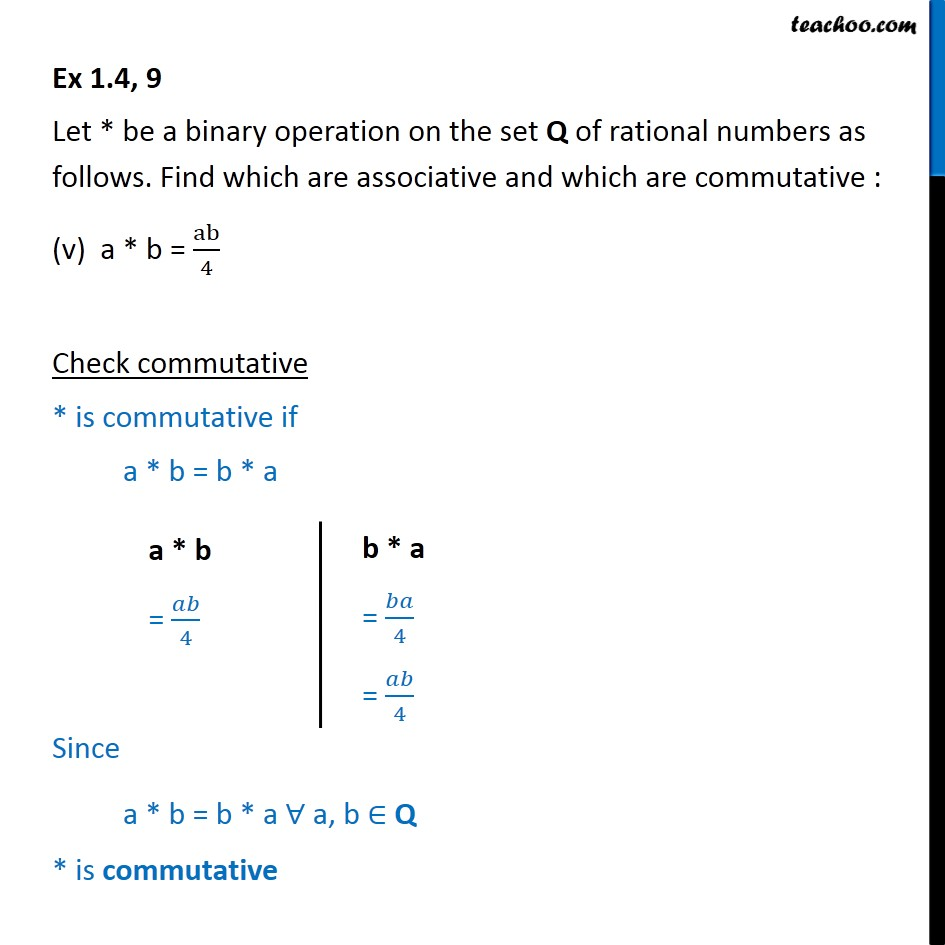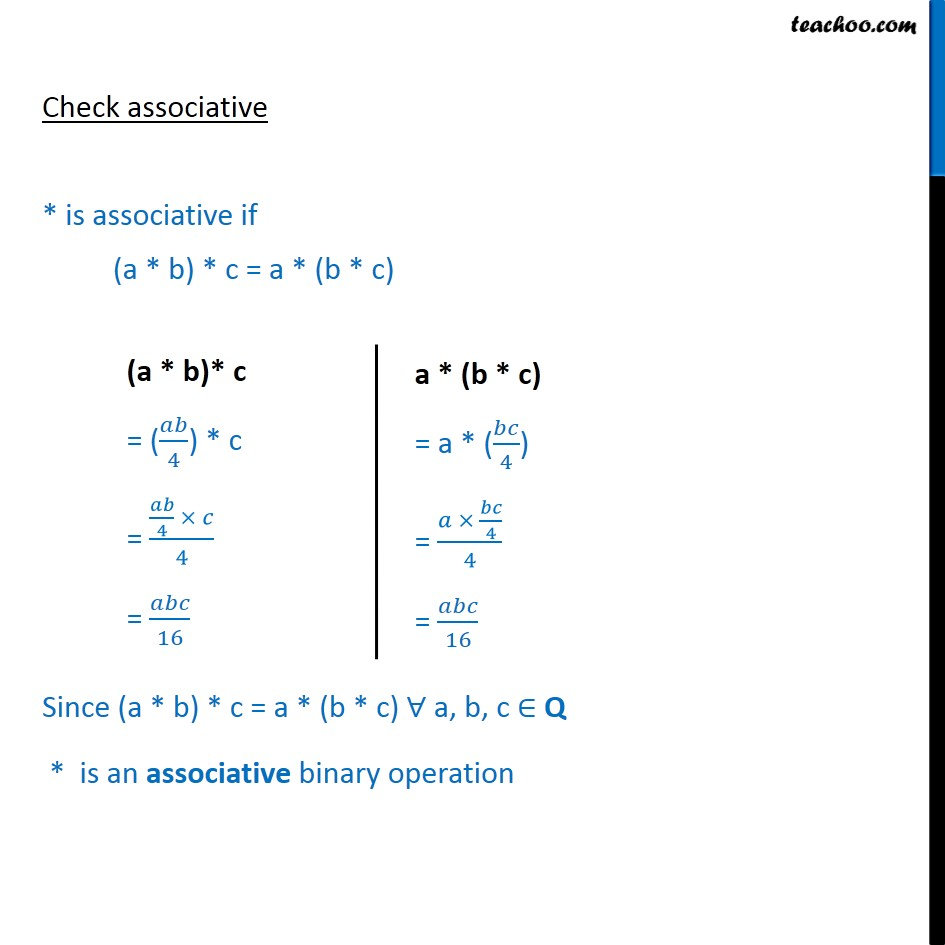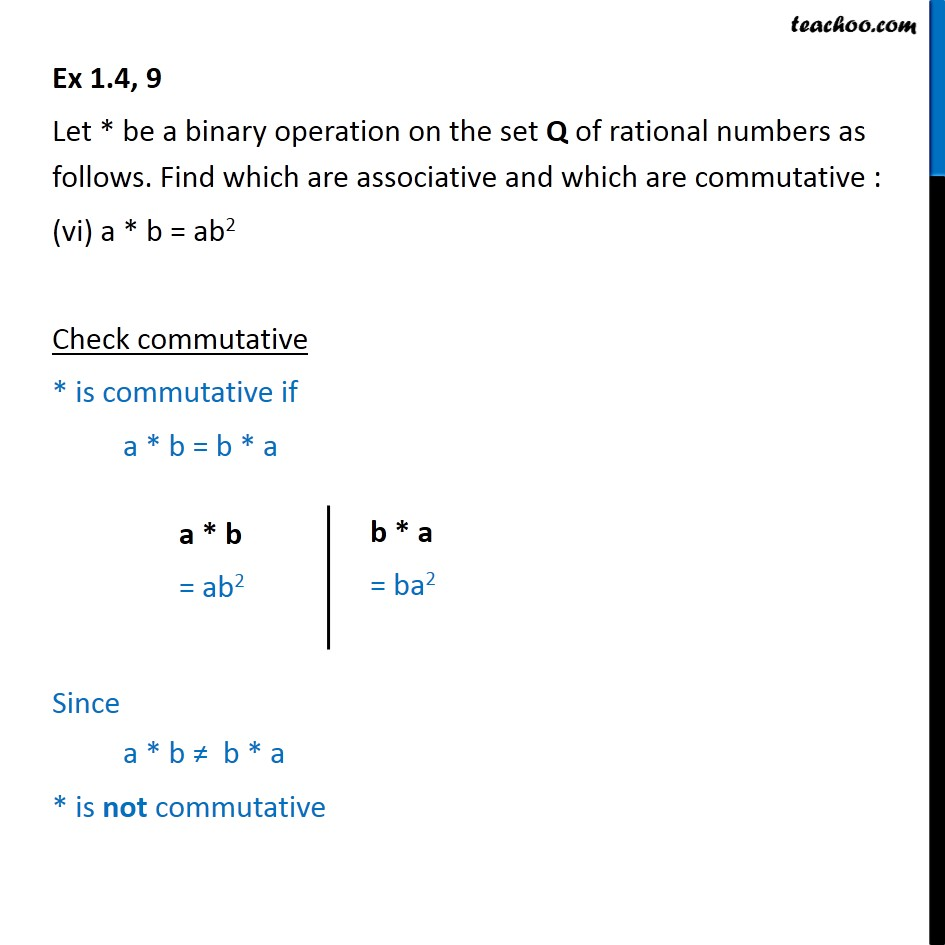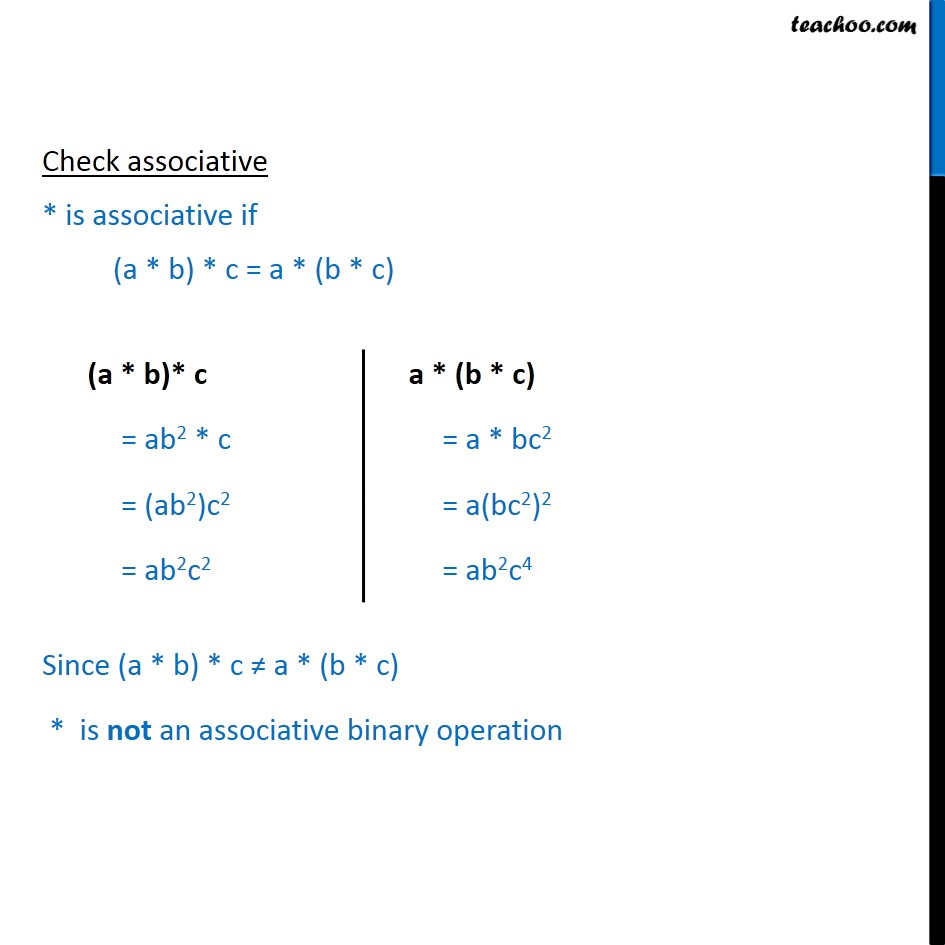Subscribe to our Youtube Channel - https://you.tube/teachoo

1. Chapter 1 Class 12 Relation and Functions
2. Serial order wise
3. Ex 1.4

Transcript

Ex 1.4, 9 Let * be a binary operation on the set Q of rational numbers as follows. Find which are associative and which are commutative : • a * b = a − b Check commutative * is commutative if a * b = b * a Since a * b ≠ b * a * is not commutative Check associative * is associative if (a * b) * c = a * (b * c) Since (a * b) * c ≠ a * (b * c) * is not an associative binary operation Ex 1.4, 9 Let * be a binary operation on the set Q of rational numbers as follows. Find which are associative and which are commutative : (ii) a * b = a2 + b2 Check commutative * is commutative if a * b = b * a Since a * b = b * a ∀ a, b ∈ Q * is commutative Check associative * is associative if (a * b) * c = a * (b * c) Since (a * b) * c ≠ a * (b * c) * is not an associative binary operation Ex 1.4, 9 Let * be a binary operation on the set Q of rational numbers as follows. Find which are associative and which are commutative : (iii) a * b = a + ab Check commutative * is commutative if a * b = b * a Since a * b ≠ b * a * is not commutative a * b = a + ab Check associative * is associative if (a * b) * c = a * (b * c) Since (a * b) * c ≠ a * (b * c) * is not an associative binary operation Ex 1.4, 9 Let * be a binary operation on the set Q of rational numbers as follows. Find which are associative and which are commutative : (iv) a * b = (a – b)2 Check commutative * is commutative if a * b = b * a Since a * b = b * a ∀ a, b ∈ Q * is commutative Check associative * is associative if (a * b) * c = a * (b * c) Since (a * b) * c ≠ a * (b * c) * is not an associative binary operation Ex 1.4, 9 Let * be a binary operation on the set Q of rational numbers as follows. Find which are associative and which are commutative : (v) a * b = ab﷮4﷯ Check commutative * is commutative if a * b = b * a Since a * b = b * a ∀ a, b ∈ Q * is commutative Check associative * is associative if (a * b) * c = a * (b * c) Since (a * b) * c = a * (b * c) ∀ a, b, c ∈ Q * is an associative binary operation Ex 1.4, 9 Let * be a binary operation on the set Q of rational numbers as follows. Find which are associative and which are commutative : (vi) a * b = ab2 Check commutative * is commutative if a * b = b * a Since a * b ≠ b * a * is not commutative Check associative * is associative if (a * b) * c = a * (b * c) Since (a * b) * c ≠ a * (b * c) * is not an associative binary operation

Ex 1.4